1595997900

R-square (Coefficient of Determination)

Do You want to find out how well the regression line (slope) fit the data? Well, then you are in the right place.

This blog will give you in-depth knowledge about r-square and how to calculate it. Gear up :D

Before you jump into this, make sure you have a good grasp on the Liner Regression and know how to calculate the intercept and the regression line (coefficient).

What is r-square?

It is the measurement of how close the data are from the fitted regression line (slope). In general the data closer to the regression line the better our machine learning model is.

R square explains how well the **independent variable **(input) explain the variation in the dependent variable (output).

Example:- If we get r-square as 0.85 it means 85% of the variation in the output can be explained by the input variable.

So, in a simple term higher the r-square, the more variation is explained by your input variable and hence better is your model.

Visual representation of R-square

To visually represent R-squared we scatter the values around the regression line, you can plot the fitted values by observed values.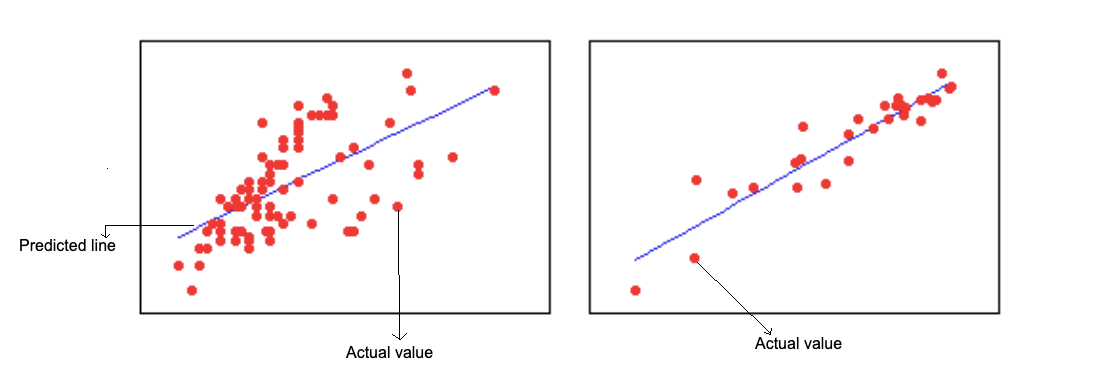The R-squared for the regression model on the left is 15%, and for the model on the right, it is 85%.

When the data points are far away from the regression line, then the r-square will be low. That means the line does not capture all the data points well.

When the data are close to the regression line, that means out model had done a good job in fitting the best line that capture all the data points well and explain all the data point well.

In practice, you’ll never see a regression model with an R2 of 100%. If it is 100%, then the predicted values will be equal to the actual value i.e. All the data will be close to the line.

How to Calculate R-square?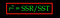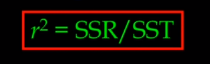Figure: r-square Formula

It is important to know

SST = SSR + SSE

Where:

**SSR (sum of square of residuals) → **Residual is the difference between the predicted value and the mean value of Y, and can be accessed by prediction model residuals.

SST (total sum of squares) → It is calculated by summing the squares of the difference between the actual value Y and the mean value of Y.

SSE (sum of the square of Error)→It is calculated by summing the square of the difference between the **actual value of Y **and predicted value.

Let’s understand this with an example, _‘Hours studied’ _as the dependent variable and ‘_Grade on Exam’ _as an independent variable.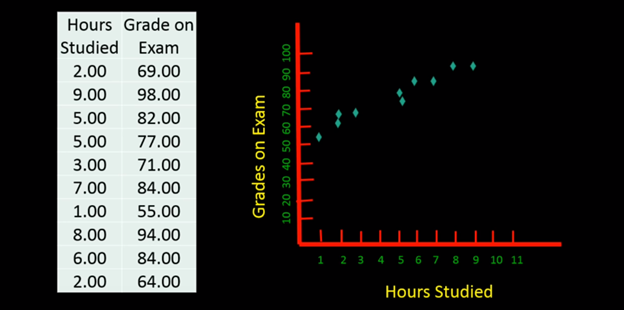Figure: Dataset and scatter plot

So, we will calculate r-square on the above Data set.

#simple-linear-regression #machine-learning #r-square #deep learning

Buddha Community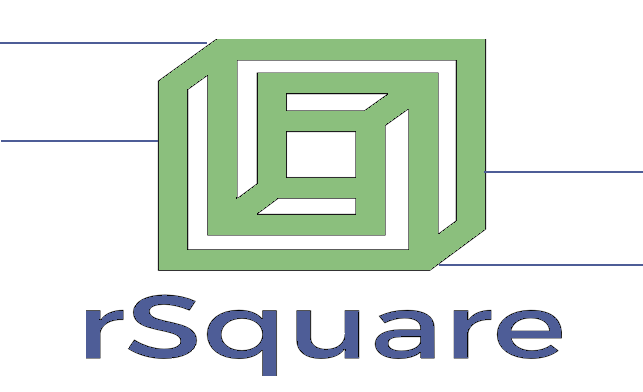1595997900

R-square (Coefficient of Determination)

Do You want to find out how well the regression line (slope) fit the data? Well, then you are in the right place.

This blog will give you in-depth knowledge about r-square and how to calculate it. Gear up :D

Before you jump into this, make sure you have a good grasp on the Liner Regression and know how to calculate the intercept and the regression line (coefficient).

What is r-square?

It is the measurement of how close the data are from the fitted regression line (slope). In general the data closer to the regression line the better our machine learning model is.

R square explains how well the **independent variable **(input) explain the variation in the dependent variable (output).

Example:- If we get r-square as 0.85 it means 85% of the variation in the output can be explained by the input variable.

So, in a simple term higher the r-square, the more variation is explained by your input variable and hence better is your model.

Visual representation of R-square

To visually represent R-squared we scatter the values around the regression line, you can plot the fitted values by observed values.The R-squared for the regression model on the left is 15%, and for the model on the right, it is 85%.

When the data points are far away from the regression line, then the r-square will be low. That means the line does not capture all the data points well.

When the data are close to the regression line, that means out model had done a good job in fitting the best line that capture all the data points well and explain all the data point well.

In practice, you’ll never see a regression model with an R2 of 100%. If it is 100%, then the predicted values will be equal to the actual value i.e. All the data will be close to the line.

How to Calculate R-square?Figure: r-square Formula

It is important to know

SST = SSR + SSE

Where:

**SSR (sum of square of residuals) → **Residual is the difference between the predicted value and the mean value of Y, and can be accessed by prediction model residuals.

SST (total sum of squares) → It is calculated by summing the squares of the difference between the actual value Y and the mean value of Y.

SSE (sum of the square of Error)→It is calculated by summing the square of the difference between the **actual value of Y **and predicted value.

Let’s understand this with an example, _‘Hours studied’ _as the dependent variable and ‘_Grade on Exam’ _as an independent variable.Figure: Dataset and scatter plot

So, we will calculate r-square on the above Data set.

#simple-linear-regression #machine-learning #r-square #deep learning1594399440

Getting Started with R Markdown — Guide and Cheatsheet

In this blog post, we’ll look at how to use R Markdown. By the end, you’ll have the skills you need to produce a document or presentation using R Mardown, from scratch!

We’ll show you how to convert the default R Markdown document into a useful reference guide of your own. We encourage you to follow along by building out your own R Markdown guide, but if you prefer to just read along, that works, too!

R Markdown is an open-source tool for producing reproducible reports in R. It enables you to keep all of your code, results, plots, and writing in one place. R Markdown is particularly useful when you are producing a document for an audience that is interested in the results from your analysis, but not your code.

R Markdown is powerful because it can be used for data analysis and data science, collaborating with others, and communicating results to decision makers. With R Markdown, you have the option to export your work to numerous formats including PDF, Microsoft Word, a slideshow, or an HTML document for use in a website.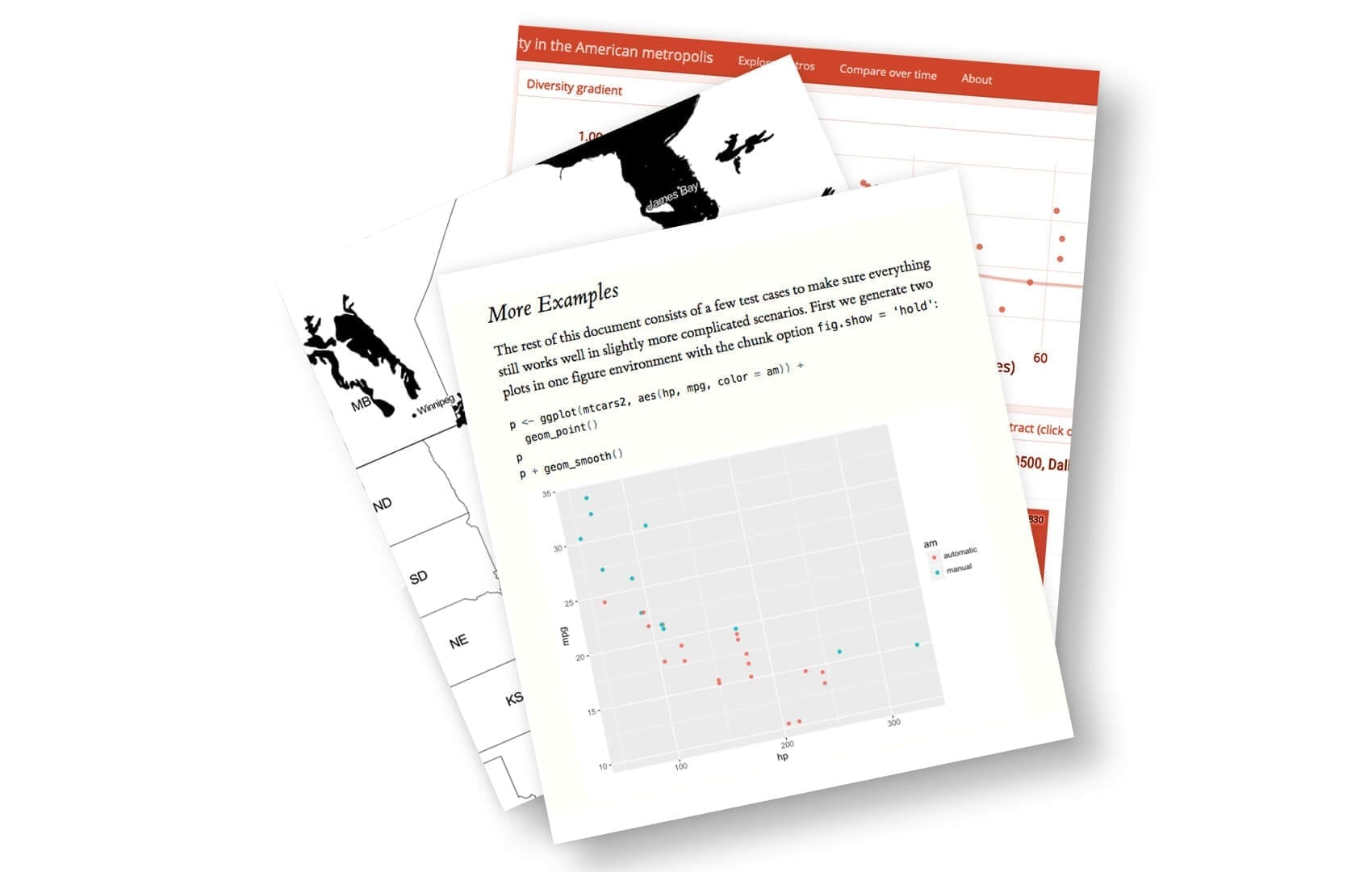Turn your data analysis into pretty documents with R Markdown.

We’ll use the RStudio integrated development environment (IDE) to produce our R Markdown reference guide. If you’d like to learn more about RStudio, check out our list of 23 awesome RStudio tips and tricks!

Here at Dataquest, we love using R Markdown for coding in R and authoring content. In fact, we wrote this blog post in R Markdown! Also, learners on the Dataquest platform use R Markdown for completing their R projects.

We included fully-reproducible code examples in this blog post. When you’ve mastered the content in this post, check out our other blog post on R Markdown tips, tricks, and shortcuts.

Okay, let’s get started with building our very own R Markdown reference document!

1. Install R Markdown

R Markdown is a free, open source tool that is installed like any other R package. Use the following command to install R Markdown:

install.packages("rmarkdown")

Now that R Markdown is installed, open a new R Markdown file in RStudio by navigating to File > New File > R Markdown…. R Markdown files have the file extension “.Rmd”.

2. Default Output Format

When you open a new R Markdown file in RStudio, a pop-up window appears that prompts you to select output format to use for the document.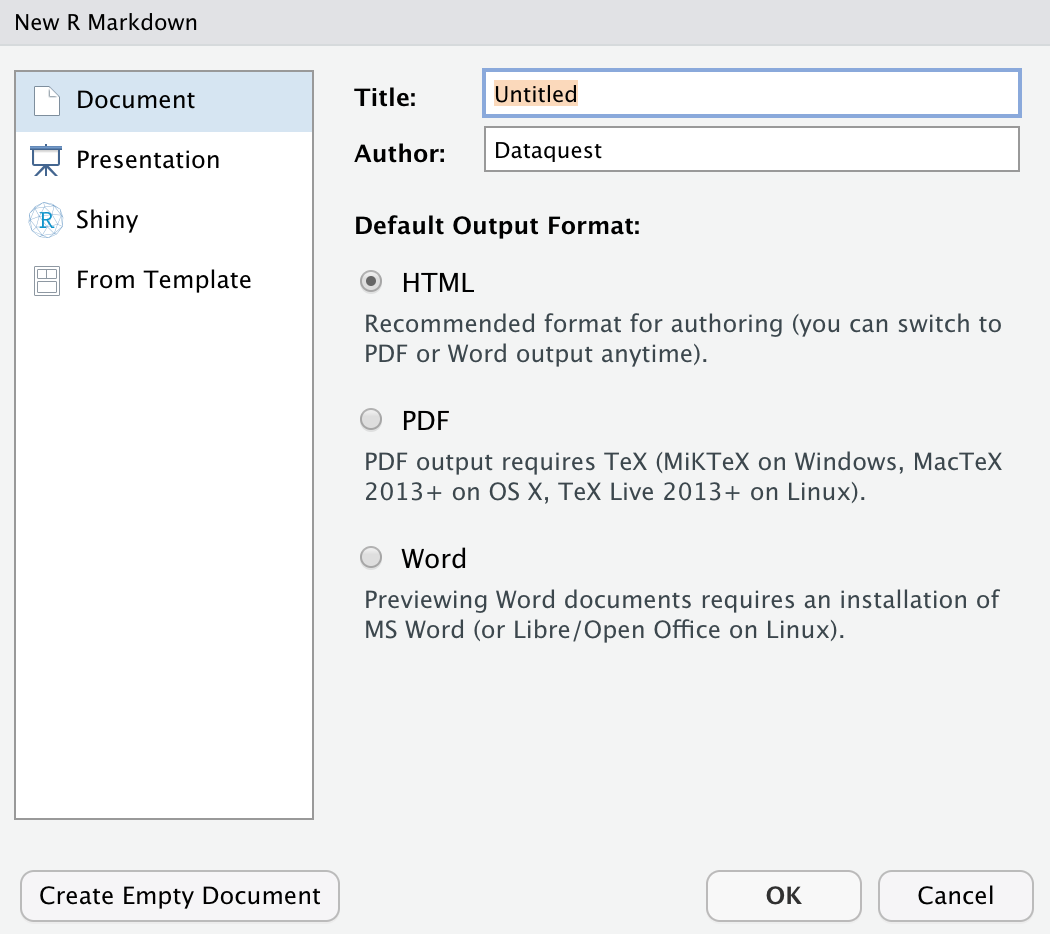The default output format is HTML. With HTML, you can easily view it in a web browser.

We recommend selecting the default HTML setting for now — it can save you time! Why? Because compiling an HTML document is generally faster than generating a PDF or other format. When you near a finished product, you change the output to the format of your choosing and then make the final touches.

One final thing to note is that the title you give your document in the pop-up above is not the file name! Navigate to File > Save As.. to name, and save, the document.

#data science tutorials #beginner #r #r markdown #r tutorial #r tutorials #rstats #rstudio #tutorial #tutorials1624422360

R vs Python: What Should Beginners Learn?

Let go of any doubts or confusion, make the right choice and then focus and thrive as a data scientist.

I currently lead a research group with data scientists who use both R and Python. I have been in this field for over 14 years. I have witnessed the growth of both languages over the years and there is now a thriving community behind both.

I did not have a straightforward journey and learned many things the hard way. However, you can avoid making the mistakes I made and lead a more focussed, more rewarding journey and reach your goals quicker than others.

Before I dive in, let’s get something out of the way. R and Python are just tools to do the same thing. Data Science. Neither of the tools is inherently better than the other. Both the tools have been evolving over years (and will likely continue to do so).

Therefore, the short answer on whether you should learn Python or R is: it depends.

The longer answer, if you can spare a few minutes, will help you focus on what really matters and avoid the most common mistakes most enthusiastic beginners aspiring to become expert data scientists make.

#r-programming #python #perspective #r vs python: what should beginners learn? #r vs python #r1611665228

R Programming Course

In this R Tutorial For Beginners video, you will learn r programming language from scratch to advance concepts. This R training video also covers hands-on demo and interview questions. This R Programming Course is a must-watch video for everyone who wishes to learn the R language and make a career in the data science domain.

#r programming course #r programming course #r tutorial for beginners #learn r language1596605640

R Markdown Tips, Tricks, and Shortcuts

R Markdown is an open-source tool for producing reproducible reports in R. It helps you keep all of your code, results, and writing in one place, and format it all in a way that’s attractive and digestible.

It’s a valuable tool for presenting your data work to others, too. With R Markdown, you have the option to export your work to numerous formats including PDF, Microsoft Word, a slideshow, or an html document for use on a website.Turn your data analysis into pretty documents with R Markdown.

R Markdown is a powerful tool because it can be used for data analysis and data science, to collaborate with others, and communicate results to decision makers.

In this blog post we’ll look at some tips, tricks, and shortcuts for working with R Markdown in RStudio. (If you’d like to learn more about RStudio, check out this Dataquest blog post for RStudio tips and tricks!)

We love using R Markdown for coding in R and authoring content. In fact, we wrote this blog post in R Markdown! Let’s check out some reasons why!

1. Keyboard Shortcuts

Knowing R Markdown keyboard shortcuts will save lots of time when creating reports.

Here are some of the essential R Markdown shortcuts:

• Insert a new code chuck with Command + Option + I on a Mac, or Ctrl + Alt + Ion Linux and Windows.
• Output your document in the format specified in your YAML header with Command + Shift + K on a Mac, or Ctrl + Shift + K on Linux and Windows. The “k” is short for “knit”!

Next we’ll cover shortcuts to run code chunks. But before doing this it is often a good idea to restart your R session and start with a clean environment. Do this with Command + Shift + F10 on a Mac or Ctrl + Shift + F10 on Linux and Windows.

• Run all chunks above the current chunk with Command + Option + P on a Mac; Ctrl + Alt + P on Linux and Windows.
• Run the current chunk with Command + Option + C or Command + Shift + Enteron a Mac; Ctrl + Alt + C or Ctrl + Shift + Enter on Linux and Windows.
• Run the next chunk with Command + Option + N on a Mac; Ctrl + Alt + N on Linux and Windows.
• Run all chunks with Command + Option + R or Command + A + Enter on a Mac; Ctrl + Alt + R or Ctrl + A + Enter on Linux and Windows.